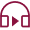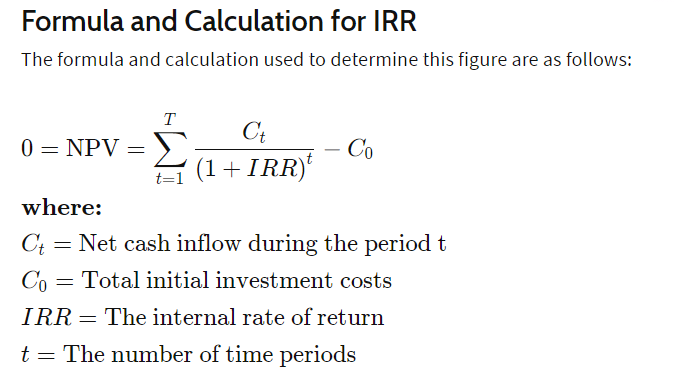Mutual Funds
Last Updated: 2023-02-06T16:15:58+05:30## Introduction

XIRR, or "internal rate of return", is a metric that shows the profitability of an investment. The internal rate of return is the discount rate that makes the series of cash flows net out to zero, which means it's the interest rate that makes the present value of all future cash flows equal to the initial investment.

The XIRR is used frequently in capital budgeting, and it's based on constant-amount cash flow streams. It relies on an accurate estimate of the future cash flow, so the internal rate of return is not appropriate for forecasting purposes. The internal rate of return provides information about profitability, not about timing.

## What is XIRR in Mutual Funds?

The internal rate of return (XIRR) tells us the interest on our investment if we had to reinvest all our earnings at this same interest rate. It would be like having to pay interest even though you did not borrow anything.XIRR is a function that calculates the internal rate of return of a security or portfolio.

XIRR takes monthly cash flows and returns and works out the annualised percentage of the cash flow value. It is helpful for investors looking to compare their mutual fund investments and can be used to calculate taxes on investments.

## XIRR in Mutual Funds-Fundamentals

XIRR is a tool mutual fund investors use to help figure out the rate of return on investment.

XIRR stands for "Internal Rate of Return". It is used to find the exact return on an investment based on compounding. You can use this tool to determine whether or not you are getting the best possible return on your investments.

It is important because it allows you to calculate your rate of return instead of just trying to estimate it. If you are using XIRR for this purpose, you will need to know how long ago your initial investment was made. You should also know exactly how much money you put into the investment initially and at what interest rate you borrowed cash if applicable. By doing this, you will see precisely how much you have gained or lost over time.

It can also be used in peer-to-peer lending (Lending Club, Prosper). Enter the amount that was lent out (loan principal), the interest rate charged by the platform, and the length of time it was lent out for (term). Then XIRR will give you back your return on investment (ROI) for that loan.

## How can you use XIRR for investments?

XIRR or Internal Rate of Return is the time-weighted rate of return on investment. It is similar to an interest rate but with more weight given to recent years.

XIRR is calculated on a series of cash flows over different periods. The first step is to find the simple interest rate for each period. The next step is to find the sum of the present values of each period's cash flows.

You can use XIRR if you know all the cash inflows and outflows over the life of an investment (including the terminal value). This is called discrete cash flow valuation or DCF or net present value.

XIRR can be used in portfolio management, capital budgeting, and my personal favourite, valuation analysis.

With XIRR, you do not need all the information with discounted cash flow (DCF). You only need an assumed growth rate and the initial investment amount.

## How do you calculate XIRR?

The formula is: XIRR = (Sum of All Cashflows from a Portfolio – Sum of all Outflows from a Portfolio)/(Number of Periods)XIRR may seem like a complicated formula, but it's not bad. You can even use Microsoft Excel's IRR function to calculate it for you.

XIRR is the Internal Rate of Return or IRR for short, one of the most important concepts in finance.

## How is XIRR a helpful metric in mutual fund investments?

XIRR is used to calculate the performance of a portfolio of investments. XIRR is calculated using Excel only and is based on an investment portfolio's cash flows (money in and money out). The period for this calculation is usually monthly, but it can be daily if you use daily cash flows.

It is a metric used to measure the rate of return on an investment that contains positive and negative cash flows. XIRR represents a more accurate estimate of the actual return on a mutual fund investment than the commonly used IRR, which only considers positive cash flows.

The metric can be calculated using either a monthly or daily timeframe. By default, a monthly timeframe is used, but this can be changed using the drop-down menu in the XIRR field. The XIRR term is typically used as an alternative to IRR as many investors find it more intuitive and accurate regarding their mutual fund returns.

## Different ways to determine XIRR

There are several ways to calculate rates of return when it comes to investing in mutual funds. It is possible to track the overall value of your investment over time by calculating an average speed of recovery. However, if you want to know exactly how much your investment has grown or shrank at any point in time, then knowing the XIRR is essential.

The XIRR is calculated using the internal rate of return formula. The IRR formula calculates the amount of money made or lost on investment between two time periods. The IRR will always be a percentage, and it can be used to calculate profit or loss for each period during an investment.

This metric allows for more calculations than just profit and loss because it focuses on the actual dollar amounts rather than percentages. The XIRR is calculated by taking the return percentage and adding it to the last dollar amount returned from a previous period while subtracting it from the first dollar amount returned from a subsequent period. Using this method will have a figure representing all actual returns instead of a percentage that can be misleading if large numbers are involved.

## XIRR in mutual funds

XIRR is an Internal Rate of Return (IRR) for mutual funds.

Mutual fund companies use internal rates of return to estimate the performance of a mutual fund over a given period. They are usually used to estimate the future returns or performance of an existing portfolio.

XIRR is calculated by first calculating the internal rate of return, then estimating the cash flows daily, and finally taking the time value of money into an account at each period.

XIRR is an Investment Performance Measurement of the Average Return of an Investment over a set period.

XIRR is used to determine the Internal Rate of Return (IRR) of an investment over a particular period. The Internal Rate of return (IRR) is the discount rate that makes the Net Present Value (NPV) of all cash flows from an investment equal to zero.

It is applied to investments that do not necessarily have cash flows in an exact time frame, like equity mutual funds.

## Wrapping Up

The importance of XIRR lies in the fact that it helps an investor understand how well a fund manager's returns have been performing against the benchmark index over a specific period.

Open Free Demat Account
Resend OTP